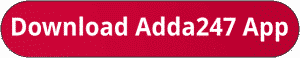Latest Banking jobs   »   IBPS RRB quant

# Quantitative Aptitude Quiz For IBPS RRB PO/Clerk Prelims 2023 -15th July

Directions (1-5): In each of the following questions, two equations (I) and (II) are given, you have to solve both the equations and give answer.
(a) If x > y
(b) If x ≥ y
(c) If x < y
(d) If x ≤ y
(e) If x = y or no relation can be established between x and y.Directions (6-10): Simplify the following equations and find the value of (?) question mark?Q7. 52703+41297-58000=100×?
(a) 720
(b) 504
(c) 360
(d) 704
(e) 840Q9. 2744÷28÷14+42=7?
(a) 1
(b) 2
(c) 0.5
(d) 1.5
(e) 3

Q10. 264÷24+190÷5=?÷5
(a) 235
(b) 305
(c) 255
(d) 245
(e) 205

Solutions. .                                                  .

.                                                        .                                                                            .                                                                              .                                                     .              .

## FAQs

### What is the selection process of IBPS RRP PO?

The Selection Process of IBPS RRB PO consists of Prelims, Mains and Interview

#### Congratulations!Union Budget 2023-24: Free PDF